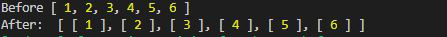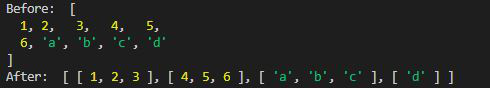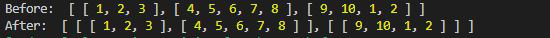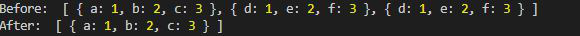# Lodash _.chunk() Method

• Last Updated : 28 Jun, 2022

Lodash is a JavaScript library that works on the top of underscore.js. Lodash helps in working with arrays, strings, objects, numbers etc. Lodash.chunk() function is used to break the array in to small chunks. Each chunk is an array of size as given.

Syntax:

`chunk(array, size)`

Parameters: This function accepts two parameters as mentioned above and describe below.

• array: An array to be processed by chunk function.
• size: This describes the size of chunk.

Return Value: It returns the array of chunks that is also an array

Note: Please install lodash module by using command npm install lodash before using the code given below.

Example 1:

## Javascript

 `// Requiring the lodash module` `// in the script` `const _ = require(``"lodash"``);` `let arr = [1, 2, 3, 4, 5, 6];`   `// Making chunks of size 1` `console.log(_.chunk(arr, 1))`

Output:OUTPUT EXAMPLE 1

Example 2: The size of chunk can be varied and array of different data type can be used with chunk function.

## Javascript

 `// Requiring the lodash module` `// in the script` `let _ = require(``"lodash"``);` `let arr = [1, 2, 3, 4, 5, 6, ` `        ``"a"``, ``"b"``, ``"c"``, ``"d"``];` `console.log(``"Before: "``, arr)`   `// Making chunks of size 3` `console.log(``"After: "``, _.chunk(arr, 3))`

Output:Example 3: Using array of array with chunk.

## Javascript

 `// Requiring the lodash module ` `// in the script.` `let lodash = require(``"lodash"``);` `let arr = [` `    ``[1, 2, 3], ` `    ``[4, 5, 6, 7, 8], ` `    ``[9, 10, 1, 2]` `];`   `console.log(``"Before: "``, arr)` `console.log(``"After: "``, lodash.chunk(arr, 2))`

Output:Example 4: Using array of Objects with chunk.

## Javascript

 `let lodash = require(``"lodash"``);` `let arr = [` `    ``{ ``"a"``: 1, ``"b"``: 2, ``"c"``: 3 }, ` `    ``{ ``"d"``: 1, ``"e"``: 2, ``"f"``: 3 }, ` `    ``{ ``"d"``: 1, ``"e"``: 2, ``"f"``: 3 }` `];`   `// Array before breaking in to chunks` `console.log(``"Before: "``, arr)`   `// Printing the first element ` `// of the chunk as size 1` `console.log(``"After: "``, ` `    ``lodash.chunk(arr, 1));`

Output :My Personal Notes arrow_drop_up
Recommended Articles
Page :